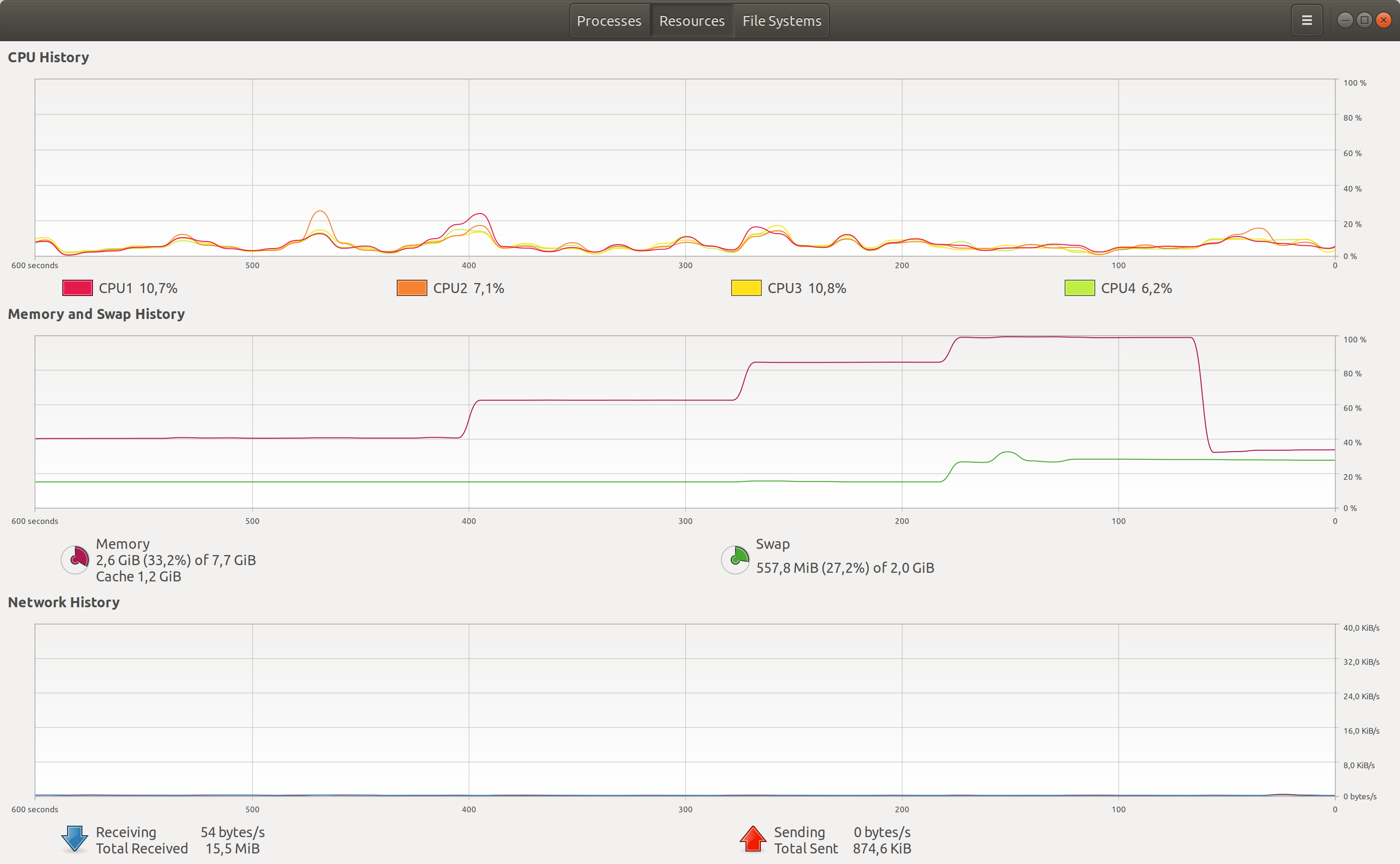# Revision history [back]

### litle code which produce memory overflow

HI

UBUNTU 18.04 Sagemath 9.1 notebook.

just for information:

this little code saturates my computer memory if executed 3 or 4 times. if I run it 2 times the memory goes up 2 times and if I reset the kernel the occupied memory goes back down. if I saturate the PC memory by executing this code 3 or 4 times then my PC becomes unusable and I have to turn off my PC and turn it back on.

import sys
import matplotlib.pyplot as plt
import matplotlib.image as mpimg
import numpy as np
from matplotlib.pyplot import figure
figS=100
figure(num=None, figsize=(figS, 2*figS), dpi=150, facecolor='w', edgecolor='k')


#### litle code which produce memory overflow

HI

UBUNTU 18.04 Sagemath 9.1 notebook.

just for information:

this little code saturates my computer memory if executed 3 or 4 times. if I run it 2 times the memory goes up 2 times and if I reset the kernel the occupied memory goes back down. if I saturate the PC memory by executing this code 3 or 4 times then my PC becomes unusable and I have to turn off my PC and turn it back on.

import sys
import matplotlib.pyplot as plt
import matplotlib.image as mpimg
import numpy as np
from matplotlib.pyplot import figure
figS=100
figure(num=None, figsize=(figS, 2*figS), dpi=150, facecolor='w', edgecolor='k')


#